# Accelerated life testing

Accelerated life tests are used to obtain advance information on the distribution of the life-time, also called time to failure, of engineering systems. Test units are subjected to higher than usual levels of stress or stresses like temperature, voltage, pressure, humidity, etc. The results from these tests, i.e., the observed life-time data, are used to make predictions about product life under more moderate conditions of use, called use stress.

In the following the basic statistical concepts in accelerated life testing are explained.

## Stress dependence of life-time distributions.

The dependence of the cumulative distribution function of a life-time on the applied stresswas modelled for exponentially distributed life-times, i.e.,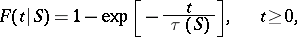by the dependence of the parameteron. For the relationship betweenand the parametercertain functionswere used with a known functionand unknown constantswhich have to be estimated from observed life-time data.

It turned out that the assumption of exponential distribution is not always justified. Therefore more general methods were needed and a non-parametric approach was developed. The formal relationship between the cumulative distribution function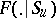of the life-time under use stress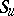and the cumulative distribution function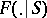of the life-time under accelerating stressis given using so-called acceleration functions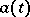by(a1)

Important forms of acceleration functions are linear acceleration functions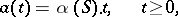(a2)

and power-type acceleration functions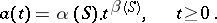(a3)

The situation of Weibull distributions (cf. Weibull distribution) with cumulative distribution function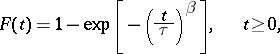and stress-dependent parametersand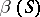is covered by power-type acceleration functions.

## Mathematical model.

The model (a1) can be applied for a one-dimensional as well as a-dimensional stress.

For linear acceleration functions and one-dimensional stress a differential equation for the acceleration factors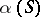can be derived. It turns out that the functionis determined by the relative acceleration constant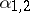inbetween two accelerating stress levels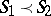.

The life-time distribution under usual stressis related toby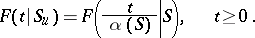For power-type acceleration functions, two differential equations forandare derived, and these functions are determined by relative acceleration constants between two accelerating stress levels. The life-time distribution under use stressis given here byFor parametric, as well as semi-parametric, models, Bayesian methods are also applied.

## Statistical inference.

Based on life-time observations on different accelerating stress levels, the following inference methods are used.

For parametric models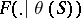, regression estimators for the constants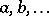in the stress dependence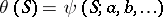of the parameter, as well as least-squares estimators, were developed.

Also, Bayesian approaches (cf. Bayesian approach) for estimating the life-time distribution under use stress are possible. Special methods for parametric, as well as for semi-parametric, accelerated life-testing models are available (compare [a1]).

Often, observations of life-times are not precise real numbers, but more or less non-precise. This imprecision is different from errors. Recently (1996), models for describing non-precise life-times and methods for accelerated life testing based on this kind of data were published (compare [a4], [a5]).